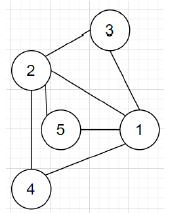# C++ program to construct graph with certain conditions

Suppose we have two numbers N and K. Consider there is an undirected graph with N elements. N vertices satisfies the following conditions −

• The graph is simple and connected

• Vertices are numbered from 1 to N

• Let M be the number of edges in the graph. The edges are numbered from 1 to M. The length of the edge is 1. And Edge i connects vertex U[i] to vertex V[i].

• There are exactly K pairs of vertices (i, j) where i < j, such that the shortest distance between them is 2.

If such graph exists, we have to construct that graph. Otherwise return -1.

So, if the input is like N = 5; K = 3, then the output will be## Steps

To solve this, we will follow these steps −

if k > (n - 1) * (n - 2) / 2, then:
print -1
print ((n - 1) * (n - 2) / 2 - k + n - 1)
for initialize i := 1, when i < n, update (increase i by 1), do:
print pair (1, i + 1)
count := (n - 1) * (n - 2) / 2 - k
for initialize i := 2, when i <= n, update (increase i by 1), do:
for initialize j := i + 1, when j <= n, update (increase j by 1), do:
if count <= 0, then:
return
print pair (i, j)
(decrease count by 1)

## Example

Let us see the following implementation to get better understanding −

#include <bits/stdc++.h>
using namespace std;

void solve(int n, int k){
if (k > (n - 1) * (n - 2) / 2){
cout << -1 << endl;
}
cout << (n - 1) * (n - 2) / 2 - k + n - 1 << '\n';
for (int i = 1; i < n; i++){
cout << 1 << ", " << i + 1 << '\n';
}
int count = (n - 1) * (n - 2) / 2 - k;
for (int i = 2; i <= n; i++){
for (int j = i + 1; j <= n; j++){
if (count <= 0){
return;
}
cout << i << ", " << j << '\n';
count--;
}
}
}
int main(){
int N = 5;
int K = 3;
solve(N, K);
}

## Input

5, 3

## Output

7
1, 2
1, 3
1, 4
1, 5
2, 3
2, 4
2, 5

Updated on: 03-Mar-2022

339 Views